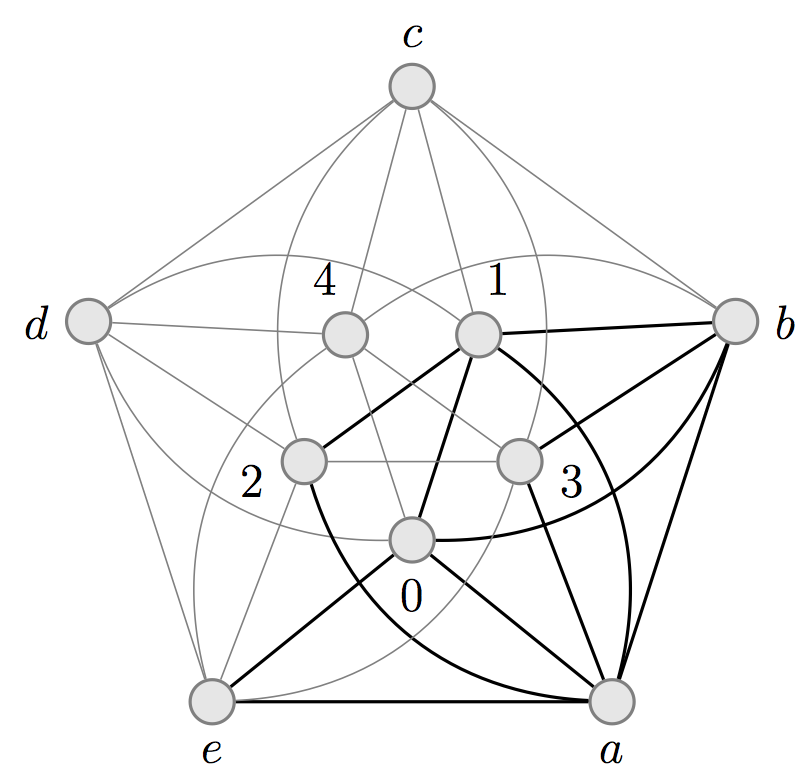# Word-representability of line graphs

### Open Journal of Discrete Mathematics, Volume 1, Number 2 (2011)A graph G=(V,E) is representable if there exists a word W over the alphabet V such that letters x and y alternate in W if and only if (x,y) is in E for each x not equal to y. The motivation to study representable graphs came from algebra, but this subject is interesting from graph theoretical, computer science, and combinatorics on words points of view. In this paper, we prove that for n greater than 3, the line graph of an n-wheel is non-representable. This not only provides a new construction of non-representable graphs, but also answers an open question on representability of the line graph of the 5-wheel, the minimal non-representable graph. Moreover, we show that for n greater than 4, the line graph of the complete graph is also non-representable. We then use these facts to prove that given a graph G which is not a cycle, a path or a claw graph, the graph obtained by taking the line graph of G k-times is guaranteed to be non-representable for k greater than 3.